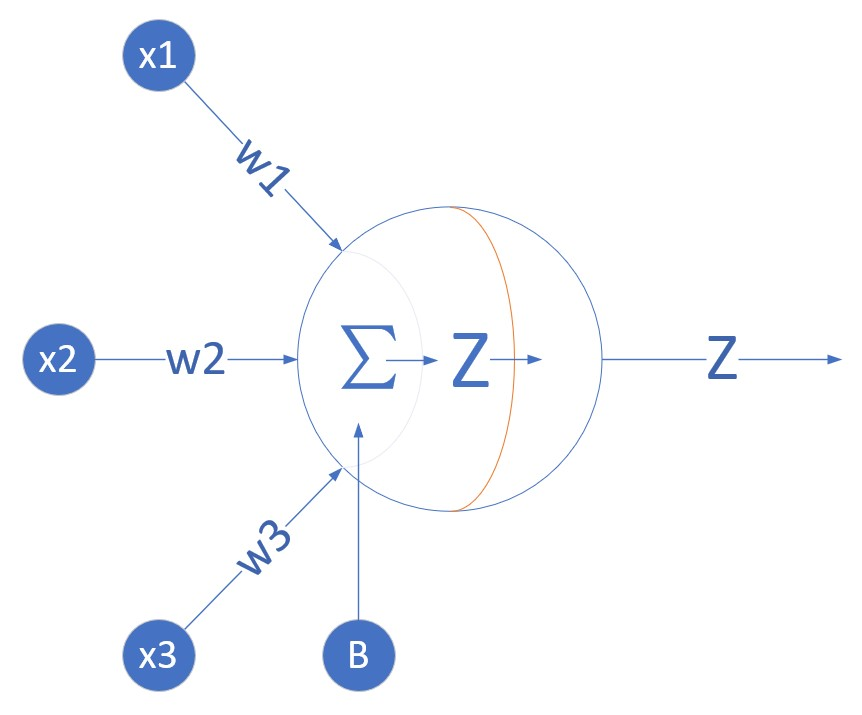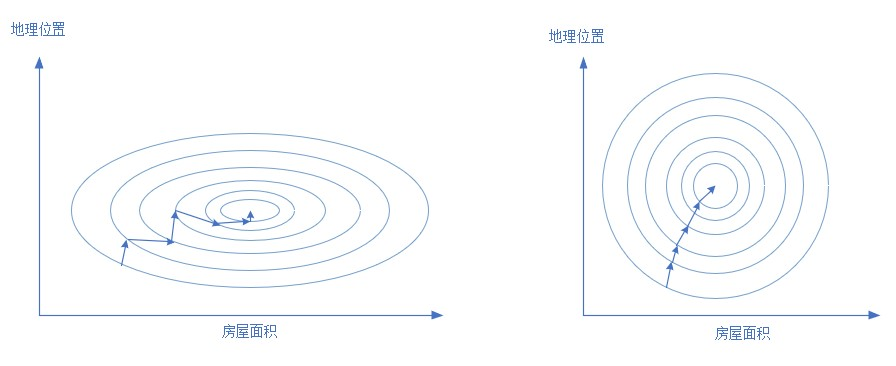# 系列之5-多入单出的一层神经网络能做什么

Copyright © Microsoft Corporation. All rights reserved.

• 多元线性回归
• 输入层的矩阵运算设计
• 样本数据归一化

# 提出问题

$y=a_0+a_1x_1+a_2x_2+\dots+a_kx_k$

$Z = w_1x_1+w_2x_2+w_3x_3+b = WX + B$

# 定义神经网络结构## 输入层

$X = \begin{pmatrix} x1_1 & x2_1 & \dots & xm_1 \\ \\ x1_2 & x2_2 & \dots & xm_2 \\ \\ x1_3 & x2_3 & \dots & xm_3 \end{pmatrix} = \begin{pmatrix} 3 & 2 & \dots & 3 \\ \\ 1 & 4 & \dots & 2 \\ \\ 96 & 100 & \dots & 54 \end{pmatrix}$

$Y = \begin{pmatrix} y1 & y2 & \dots & ym \\ \end{pmatrix}= \begin{pmatrix} 434 & 500 & \dots & 410 \\ \end{pmatrix}$

$x1 = \begin{pmatrix} x1_1 \\ \\ x1_2 \\ \\ x1_3 \end{pmatrix} = \begin{pmatrix} 3 \\ \\ 1 \\ \\ 96 \end{pmatrix}$

$y1 = \begin{pmatrix} 434 \end{pmatrix}$

$x1$表示第一个样本，$x1_1$表示第一个样本的一个特征值。

## 权重W和B

$\begin{pmatrix} w1 & w2 & w3 \end{pmatrix} \times \begin{pmatrix} x1_1 \\ \\ x1_2 \\ \\ x1_3 \end{pmatrix}+B= w1*x1_1+w2*x1_2+w3*x1_3+B$

$\begin{pmatrix}w_1 & w_2 \dots w_n\end{pmatrix}$

B是个单值，因为只有一个神经元，所以只有一个bias，每个神经元对应一个bias，如果有多个神经元，它们都会有各自的b值。

# 收集训练数据

• 朝向（在北方地区，窗户面向阳光的房子要抢手一些）：北=1，西=2，东=3，南=4
• 地理位置：二环，三环，四环，五环，六环，分别取值为2，3，4，5，6，二环的房子单价最贵
• 面积：40~120平米，连续值

import numpy as np
import matplotlib.pyplot as plt
from pathlib import Path

# y = w1*x1 + w2*x2 + w3*x3 + b
# W1 = 朝向：1,2,3,4 = N,W,E,S
# W2 = 位置几环：2,3,4,5,6
# W3 = 面积:平米
def TargetFunction(x1,x2,x3):
w1,w2,w3,b = 2,10,5,10
return w1*x1 + w2*(10-x2) + w3*x3 + b

def CheckFileData():
Xfile = Path("HouseXData.npy")
Yfile = Path("HouseYData.npy")
if Xfile.exists() & Yfile.exists():
XData = np.load(Xfile)
YData = np.load(Yfile)
return True,XData,YData

return False,None,None

def create_sample_data(m):
flag,XData,YData = CheckFileData()
if flag == False:
X0 = np.random.randint(1,5,m)
X1 = np.random.randint(2,7,m)
X2 = np.random.randint(40,120,m)
XData = np.zeros((3,m))
XData = X0
XData = X1
XData = X2
Y = TargetFunction(X0,X1,X2)
noise = 20
Noise = np.random.randint(1,noise,(1,m)) - noise/2
YData = Y.reshape(1,m)
np.save("HouseXData.npy", XData)
np.save("HouseYData.npy", YData)
return XData, YData

$z = w1·x_1 + w2·(10-x_2)+w3·x_3+b \\ = 2x_1 - 10(10-x_2)+5x_3+10 \\ = 2x_1 - 10x_2+5x_3+110$

# 定义前向计算过程

def forward_calculation(Xm,W,b):
z = np.dot(W, Xm) + b
return 

# 定义代价函数

$Loss = \frac{1}{2}(Z - Y) ^ 2$

def check_diff(w, b, X, Y, count, prev_loss):
Z = w * X + b
LOSS = (Z - Y)**2
loss = LOSS.sum()/count/2
diff_loss = abs(loss - prev_loss)
return loss, diff_loss

# 求针对W和B的梯度函数

$z = wx+b$

$loss = \frac{1}{2}(z-y)^2$

$\frac{\partial{loss}}{\partial{w}} = \frac{\partial{loss}}{\partial{z}}*\frac{\partial{z}}{\partial{w}} = (z-y)x$

$\frac{\partial{loss}}{\partial{b}} = \frac{\partial{loss}}{\partial{z}}*\frac{\partial{z}}{\partial{b}} = z-y$

def dJwb_single(Xm,Y,Z):
dloss_z = Z - Y
db = dloss_z
dw = np.dot(dloss_z, Xm.T)
return dw, db

# 每次迭代后更新W,B的值

def update_weights(w, b, dw, db, eta):
w = w - eta*dw
b = b - eta*db
return w,b

# 主程序初始化

m = 1000    # 创造1000个样本
XData, Y = create_sample_data(m)
X = XData
n = X.shape  # 每个样本的特征数
eta = 0.1   # 学习率0.1
loss, diff_loss, prev_loss = 10, 10, 5
eps = 1e-10
max_iteration = 100 # 最多100次循环
# 初始化w,b
b = np.zeros((1,1))
w = np.zeros((1,n))

# 程序主循环

for iteration in range(max_iteration):
for i in range(m):
Xm = X[0:n,i].reshape(n,1)
Ym = Y[0,i].reshape(1,1)
Z = forward_calculation(Xm, W, B)
dw, db = dJwb_single(Xm, Ym, Z)
W, B = update_weights(W, B, dw, db, eta)

loss, diff_loss = check_diff(w,b,xxx,y,1,prev_loss)
if diff_loss < eps:
print(i)
break
prev_loss = loss
print(iteration, w, b, diff_loss)
if diff_loss < eps:
break

C:\Users\Python\LinearRegression\MultipleInputSingleOutput.py:61: RuntimeWarning: overflow encountered in square
LOSS = (Z - Y)**2
C:\Users\Python\LinearRegression\MultipleInputSingleOutput.py:63: RuntimeWarning: invalid value encountered in double_scalars
diff_loss = abs(loss - prev_loss)
C:\Users\Python\LinearRegression\MultipleInputSingleOutput.py:55: RuntimeWarning: invalid value encountered in subtract
w = w - eta*dw
0 [[nan nan nan]] [[nan]] nan
1 [[nan nan nan]] [[nan]] nan
2 [[nan nan nan]] [[nan]] nan
3 [[nan nan nan]] [[nan]] nan

# 解决梯度爆炸

## 检查迭代中的数值变化情况

0 -----------
Z: [[0.]]
Y: []
dLoss/dZ: [[-469.]]
dw: [[  -938.  -1876. -37051.]]
db: [[-469.]]
W: [[  93.8  187.6 3705.1]]
B: [[46.9]]
1 -----------
Z: [[289982.7]]
Y: []
dLoss/dZ: [[289518.7]]
dw: [[  579037.4         1158074.8        22582458.60000001]]
db: [[289518.7]]
W: [[  -57809.94  -115619.88 -2254540.76]]
B: [[-28904.97]]
2 -----------
Z: [[-2.62364972e+08]]
Y: []
dLoss/dZ: [[-2.62365606e+08]]
dw: [[-5.24731213e+08 -1.57419364e+09 -3.04344103e+10]]
db: [[-2.62365606e+08]]
W: [[5.24153113e+07 1.57303744e+08 3.04118649e+09]]
B: [[26207655.65900001]]
......

0 ----------
Z: [[0.]]
Y: []
dLoss/dZ: [[-469.]]
dw: [[  -938.  -1876. -37051.]]
db: [[-469.]]
W: [[ 0.938  1.876 37.051]]
B: [[0.469]]
1 -----------
Z: [[2899.827]]
Y: []
dLoss/dZ: [[2435.827]]
dw: [[  4871.654   9743.308 189994.506]]
db: [[2435.827]]
W: [[  -3.933654   -7.867308 -152.943506]]
B: [[-1.966827]]
2 ----------
Z: [[-17798.484679]]
Y: []
dLoss/dZ: [[-18432.484679]]
dw: [[  -36864.969358  -110594.908074 -2138168.222764]]
db: [[-18432.484679]]
W: [[  32.93131536  102.72760007 1985.22471676]]
B: [[16.46565768]]

## 数据归一化

### min-max标准化

$x_{new} = \frac{x-x_{min}}{x_{max}-x_{min}}$

$x_{new} = \frac{x-x_{mean}}{x_{max}-x_{min}}$
mean表示数据的均值。

### 样本分析

• 特征值1 - 窗户朝向
一共有”东”“南”“西”“北“四个值，用数字化表示是：
东：3
南：4
西：2
北：1
因为超南的房子比较贵，其次为东，西，北。为啥东比西贵？因为夏天时朝西的窗户西晒时间长，比较热。

• 特征值2 - 地理位置
二环：2 - 单价最贵
三环：3
四环：4
五环：5
六环：6 - 单价最便宜

• 特征值3 - 房屋面积
统计所有样本数据得到房屋的面积范围是[40,120]

### 数据归一化的好处### 归一化的实现

def Normalize(X):
X_new = np.zeros(X.shape)
n = X.shape
w_num = np.zeros((1,n))
for i in range(n):
v = X[i,:]
max = np.max(v)
min = np.min(v)
w_num[0,i] = max - min
v = (v - min)/(max-min)
X_new[i,:] = v
return X_new, w_num

m = 1000
XData, Y = create_sample_data(m)
X, W_num = Normalize(XData)
n = X.shape
eta = 0.1
loss, diff_loss, prev_loss = 10, 10, 5
eps = 1e-10
max_iteration = 1

B = np.zeros((1,1))
W = np.zeros((1,n))

for iteration in range(max_iteration):
for i in range(m):
Xm = X[0:n,i].reshape(n,1)
Ym = Y[0,i].reshape(1,1)
Z = forward_calculation(Xm, W, B)
dw, db = dJwb_single(Xm, Ym, Z)
W, B = update_weights(W, B, dw, db, eta)
loss, diff_loss = check_diff(W,B,Xm,Ym,1,prev_loss)
if diff_loss < eps:
print(i)
break
prev_loss = loss
print(iteration, W, B, diff_loss)
if diff_loss < eps:
break

[[ 5.94417016 -40.05847929 394.83396979]] [[292.15951172]]

$w1=5.94417016 \\ w2=-40.05847929 \\ w3=394.83396979 \\ b=292.15951172$

$z = w1·x_1 + w2·(10-x_2)+w3·x_3+b \\ = 2x_1 - 10(10-x_2)+5x_3+10 \\ = 2x_1 - 10x_2+5x_3+110 \\ w1=2 \\ w2=-10 \\ w3=5 \\ b=110$

# 归一化的后遗症

w1是窗户朝向，取值范围[1,4]，即：$x_{min}=1, x_{max}=4$，目前$w1=5.94417$，试着变换一下：

$\frac{w1}{x_{max}-x_{min}} = \frac{5.94417}{4-1}=1.98139 \simeq 2$

$\frac{w2}{x_{max}-x_{min}} = \frac{-40.058}{6-2}=-10.0145 \simeq -10$

$\frac{w3}{x_{max}-x_{min}} = \frac{394.8339}{119-40}=4.997 \simeq 5$

W_rel = np.zeros((1,n))
for i in range(n):
W_rel[0,i] = W[0,i] / W_num[0,i]
print(W_rel)

“卑职以为，b值不是样本值，没有经过归一化。”

“你说得很对！但我们怎么解出b呢？”

“大人，这个好办，我们如此这样这样......把一堆样本值代入w1,w2,w3的公式，令b=0，看看计算出来的结果是什么，再和样本标签值去比较就可以了！”
$z = w1*x_1+w2*x_2+w3*x_3 +0\\ b = y - z$

B = 0
for i in range(10):
xm = XData[0:n,i].reshape(n,1)
zm = forward_calculation(xm, W_rel, 0)
ym = Y[0,i].reshape(1,1)
B = B + (ym - zm)
b = B / 10
print(b)

[[  1.98139005 -10.01461982   4.99789835]]
[[110.29389945]]posted @ 2018-12-03 12:09 UniversalAIPlatform 阅读(...) 评论(...) 编辑 收藏